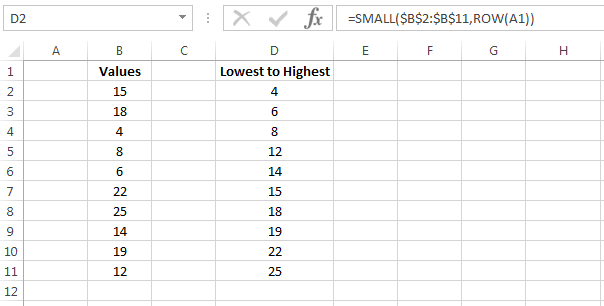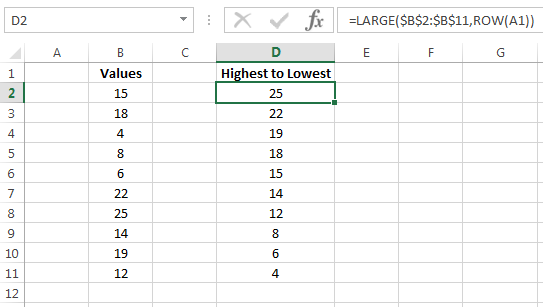Our Recent Posts

Archive

Tags

No tags yet.

# Sort with a Formula in Excel

Excel has a built in sort feature which enables you to sort data in ascending or descending order. There are alternative ways to sort data in Excel. I am going to show you how to sort with a formula in Excel by using the Excel LARGE and SMALL functions.

### Sorting Data from Lowest to HighestI have a list of values in column B and I want to sort this from the lowest value through to the highest value. In order to sort this I will use the Excel SMALL function. Before I explain how to do this it is important to understand what the SMALL function does.

### What is the Excel SMALL Function?

The Excel SMALL function returns the nth smallest value in a given list. For example, if I want the second smallest value in the range 10, 25, 5, 8 the SMALL function will return 8 as 8 is the second smallest value in the list.

SMALL Function Argumentsarray – This is a required argument and is the range of cells where you want to extract the nth smallest number

n – This is a required argument and is the specified smallest value you want to extract from the arrayThe formula in cell D2 is =SMALL(\$B\$2:\$B\$11,ROW(A1)). The array argument is the cell range B2:B11. I have used the ROW function as the n argument. The ROW function simply returns the row number for a cell reference. For example, cell A1 will return 1 as A1 is in row 1. B4 will return 4, E9 will return 9 and so on. The formula in cell D2 returns the lowest number as the n argument is row A1.

Notice the array argument B2:B11 is absolute as they have dollar signs before the column and row. The n argument is relative. This is because when I copy the formula down I want to keep the array argument the same but I want the n argument to change each time. For example, when I copy the formula down to cell D3 the ROW function will change to cell A2 and so therefore it will extract the second lowest value. For cell D4 the ROW function will change to A3 and will therefore return the third lowest value and so on.This is what the formulas look like when I copy them down in column D. To learn how to show formulas then please see my tutorial on How to Show Formulas in Excel. Notice the ROW function increments by one after each row which will give me the nth value.

You can of course hard code the nth value but this is a painful task, especially if you have many rows of data.

### Sorting Data from Highest to LowestI now want to sort the data in the cell range B2:B11 from highest to lowest. This is very similar to using the SMALL function but you need to instead use the LARGE function. I will first explain what the LARGE function is.

### What is the LARGE Function?

The LARGE function will return the nth largest value in a given list. For example, if I want the second largest value in the range 10, 25, 5, 8 the LARGE function will return 10 as 10 is the second largest value in the list.

LARGE Function Argumentsarray – This is a required argument and is the range of cells where you want to extract the nth largest number

n – This is a required argument and is the specified largest value you want to extract from the arrayThe formula in cell D2 is =LARGE(\$B\$2:\$B\$11,ROW(A1)). The array argument is the cell range B2:B11. I have used the ROW function as the n argument. This works in exactly the same way as the SMALL function with the only difference being the LARGE function sorts the data from largest to lowest.

I hope you have enjoyed this tutorial on how to sort with a formula in Excel. If you have any questions, feedback or know of any other ways to sort data then please leave me a comment below.